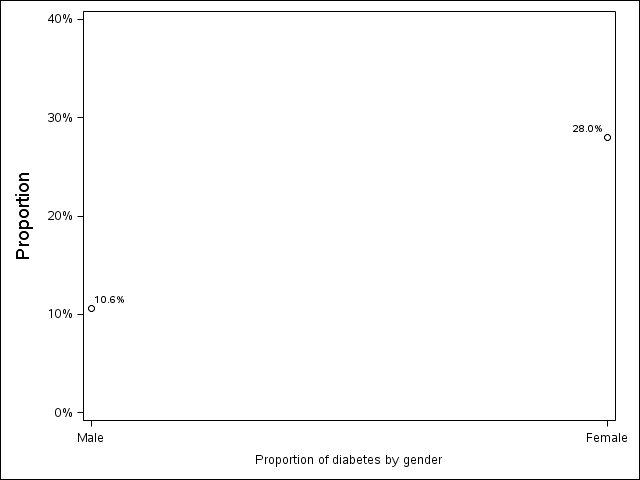## How to display values for ticks on axis as percentage (e.g. 8%) in a scatter plot

Dear all,

Does anyone know how to specify the value for ticks on axis as percentage (e.g. '8%') instead of number (e.g. '8') for a scatter plot ?

I used 'Proc sgplot' to create a scatter plot for proportion of diabetes in male and female. The Y axis is proportion and I want to display percentage here. Thanks a lot!

Best,

Feng Liang

1 ACCEPTED SOLUTION

Accepted Solutions

## Re: How to display values for ticks on axis as percentage (e.g. 8%) in a scatter plot

Dear JeffMeyers,

Thank you very much !

Regards,

Feng Liang

6 REPLIES 6

## Re: How to display values for ticks on axis as percentage (e.g. 8%) in a scatter plot

Could you please send the proc sgplot code you are using. Before that you might try using the proc format as below

proc format;
value pct
low - 1='1%'
2-<3='2%'
3-<4='4%';
run;

you can extend the format. then you need to apply this format in the sgplot procedure for the y axis variable.
Thanks,
Jag

## Re: How to display values for ticks on axis as percentage (e.g. 8%) in a scatter plot

Dear ,

The following are my codes:

## Re: How to display values for ticks on axis as percentage (e.g. 8%) in a scatter plot

Sorry, the codes were not posted properly. I post them again.

data pdi;
INPUT Gender \$ Proportion;
cards;
Male    10.6
Female  28.0

;
RUN;

PROC SGPLOT DATA=pdi;
SCATTER Y=Proportion X=gender/ datalabel=proportion ;
YAXIS VALUES=(0 TO 40 BY 10) LABEL="Proportion " LABELATTRS=(size=13 );
XAXIS LABEL="Proportion of diabetes by gender"  ;
RUN;QUIT;

Thanks!

Feng Liang

## Re: How to display values for ticks on axis as percentage (e.g. 8%) in a scatter plot

In that case you coudl try something as below

``````data pdi;
INPUT Gender \$ Proportion;
cards;
Male    10.6
Female  28.0
;
RUN;

proc format;
value pct
10.6='10.6%'
28.0='28.0%'
;

use this format on the variable in proc sgplot``````
Thanks,
Jag

## Re: How to display values for ticks on axis as percentage (e.g. 8%) in a scatter plot

Hello,

I would just divide your proportions by 100 and add the percent12.1 format to it.  I had to adjust the y axis values (didn't change your label yet) afterwards.  Here's what I got with your code:

`````` data pdi;
input gender \$ proportion;
cards;
Male 10.6
Female 28.0
;
run;
data pdi2;
set pdi;
proportion=proportion/100;
run;

ods graphics /reset;

proc sgplot data=pdi2;
scatter y=proportion x=gender / datalabel=proportion;
yaxis values=(0 to 0.4 by 0.1) Label='Proportion' labelattrs=(size=13);
xaxis label='Proportion of diabetes by gender';
format proportion percent12.1;
run;

``````## Re: How to display values for ticks on axis as percentage (e.g. 8%) in a scatter plot

Dear JeffMeyers,

Thank you very much !

Regards,

Feng Liang

Discussion stats
• 6 replies
• 7423 views
• 1 like
• 3 in conversation